Spatial Analysis with SQL: 4-Spatial Processing Functions

In the previous article we discussed the SQL functions of spatial relationship . Let’s now discuss the SQL spatial processing functions. Let’s start with the difference between the notion of spatial relation and spatial treatment.

The simplest way is to use an example. We have geometries that share the occupation of spaceA spatial relationship function, such as st_intersects , will answer the question “do these geometries intersect ?”  TRUE or FALSE?.

A spatial processing function, such as ST_Intersection, will extract the common part of the geometries returning as result a geometry :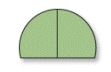Let’s suppose that we have two tables: rectangles and circles, and that we want to retrieve the result from this image. How does one design the adequate SQL query ?

By adopting the same approach as described in the second article of this series :

• which is the data I want to obtain ?: I want the resulting geometries originating in the intersection of circles and rectangles-> SELECT clause:

SELECT st_ intersection ( circles.geometry, rectangles.geometry ) as geometry

• where are they stored ? : in the circles and rectangles tables-> FROM clause:

FROM circles, rectangles

• which are the conditions I want to apply , spatial or not?  select only entities that occupy common space -> WHERE clause:

WHERE st_ intersects ( circles.geometry, rectangles.geometry )

What makes the request :

SELECT st_ intersection ( circles.geometry, rectangles.geometry ) as geometry
FROM circles, rectangles
WHERE st_intersects ( circles.geometry, rectangles.geometry )

You can omit the WHERE clause and get the same result, except that it will take a lot more time. It is logical to limit the operation intersection of st_intersection to places where the geometries intersect.

Thebatchand interactive tools

Before discussing the spatial processing functions in detail, let’s do a little parenthesis. In office GIS, such as ArcGis, you, usually, work with two groups of different tools according to what you are going to process (a layer as a whole or a specific entity).

When processing the layer as a whole, you use the tools in the Toolbox. When processing the specific entities you use the ArcMap interactive tools.

An advantage of SQL is that you work with only one tool. Let’s return to the previous query to apply it at the intersections between a layer map_ risks ( in beige)   and flooding areas ( in blue)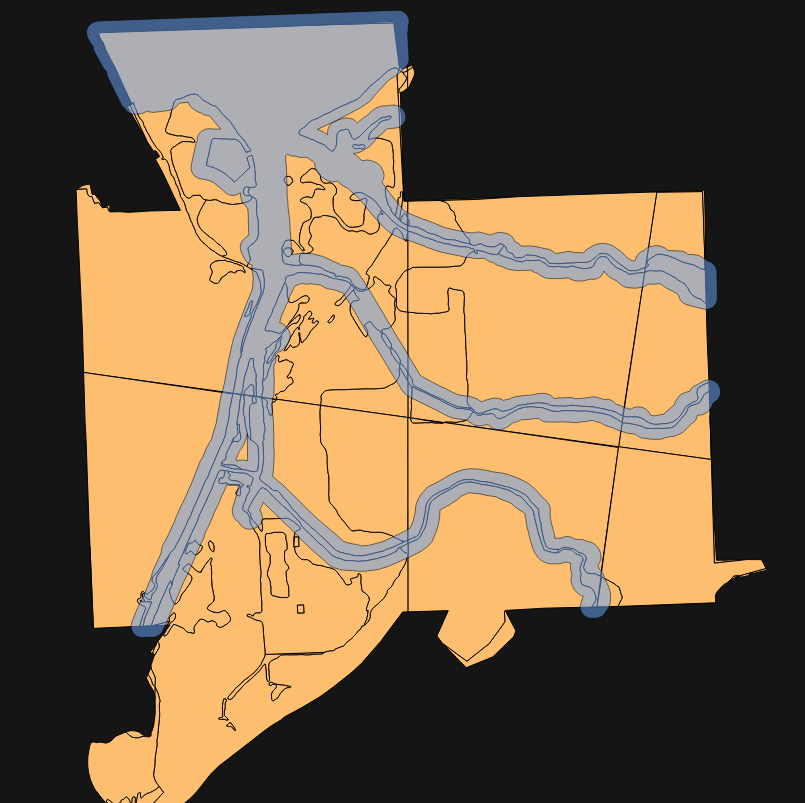We can use the query :

SELECT st_ intersection ( map_risk.geometry, flooding_zones.geometry ) as geometry
FROM map_risk, flooding_zones
WHERE st_intersects ( map_risk.geometry, flooding_zones.geometry )

To intersect all the present entities in the risk map with flooding areas: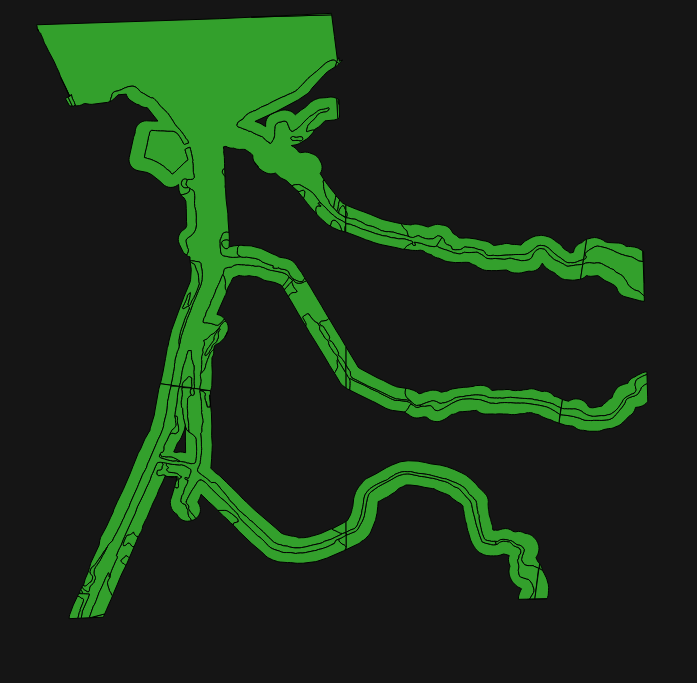Where only the area with a Maximum risk for flood areas

SELECT st_ intersection ( map_risk.geometry, flooding_zones.geometry ) as geometry
FROM map_risk, flooding_zones
WHERE st_intersects ( map_risk.geometry, flooding_zones.geometry ) AND
map_risk.zone = ‘max’The only difference is in the WHERE clause where we add ”   AND
map_risk.zone = ‘max’ «

Spatialprocessingfunctions

If you take a look to the help page of ArcGis Overlay tools ( https://pro.arcgis.com/fr/pro-app/tool-reference/analysis/an-overview-of-the-overlay-toolset.htm ) you will find a description of a series of spatial processing functions: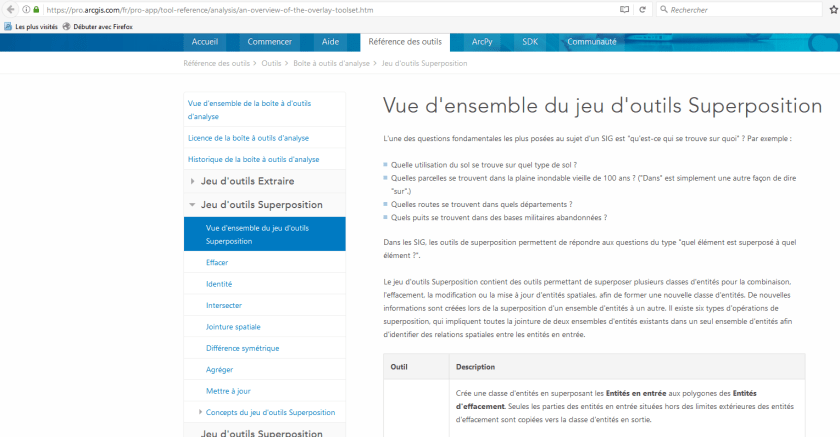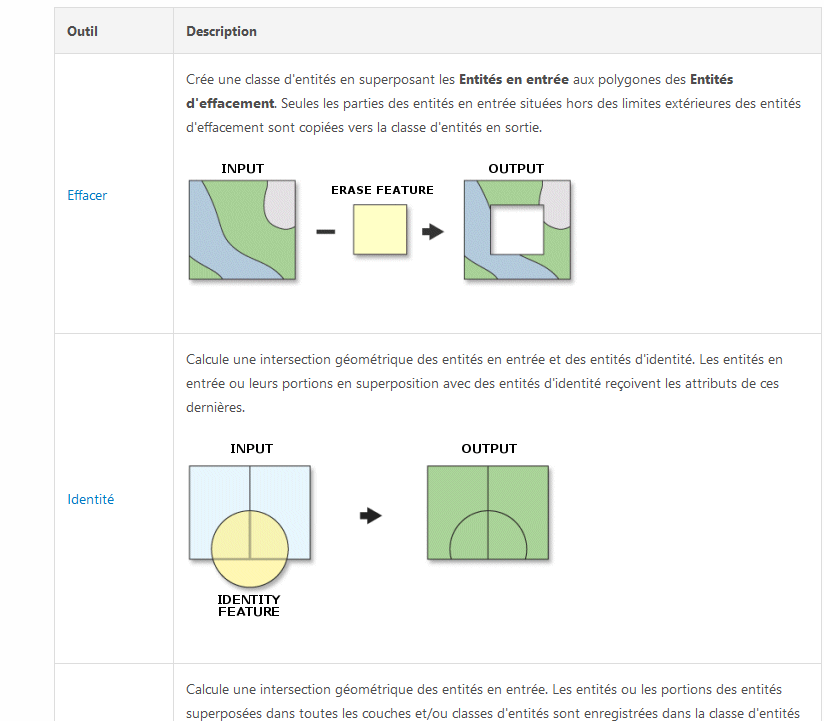They are seven: Erase, Identity, Intersection, Spatial joint, Symmetric difference, Aggregation and Update.

In SQL we will use only three functions: st_difference , st_intersection and ST_Union to build the equivalent queries.

Firstly, let’s discuss these three functions and their equivalent in the ArcGis Toolset , then we will discuss how to get the equivalence with others tools from the Toolset combining the three basic functions .

st_Difference= Erase

It bears the form st_ difference (geometry A, geometry B). This function returns the A geometry part that does not intersect the B geometry.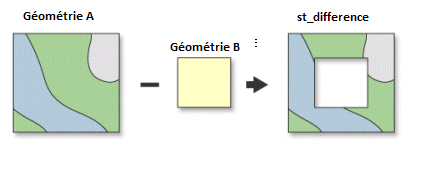In the risk mapping and flood zones example we can wish, for example, to see the part of the classified areas as maximum risk that are not floodable:

SELECT ST_ Difference (map_risk.geometry, flooding_zones.geometry) as geometry
FROM flooding_zones, map_risk
WHERE map_risk.zone = ‘max’

The result of the query appears in yellow.st_intersection= Intersection

It bears the form ST_ intersection geometry, B geometry). This function returns the part of the A geometry that intersects   the B geometry.We have already detailed the query

SELECT st_ intersection ( map_risk. geometry, flooding_zones.geometry ) as geometry
FROM map_risk, flooding_zones
WHERE st_intersects ( map_risk.geometry, flooding_zones.geometry )

in the previous paragraph dealing with batch and interactive functions. But we will complete the use of functions with another element. In the examples used so far we get as result of the query only geometries. But most of the time we, also, need to recover the attributes of the concerned entities.

For example, in the resulting entities of the query we want to know the version of   “flooding zones” as well as the risk level. We add these fields in the SELECT part of the query

SELECT flooding_zones.version , map_risk.zone , st_ intersection ( map_risk.geometry , flooding_zones.geometry ) as geometry
FROM map_risk, flooding_zones
WHERE st_intersects (map_risk.geometry , flooding_zones.geometry )

and the result will be: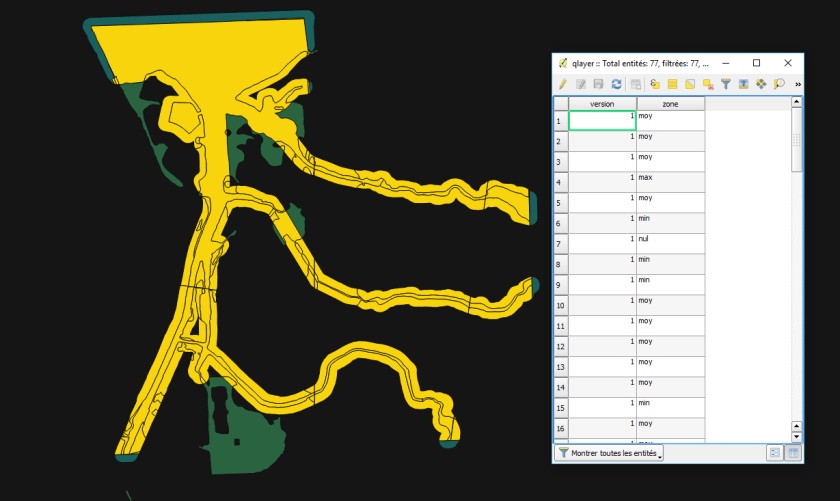Attributes fields are added in the resulting table. Each geometry will have the value of the attribute of the geometries that intersect at that place.

ST_Union

It bears the form st_ union ( A geometry, B geometry). This function take each A geometry and merging with the B geometry/es by merging the points that compose them.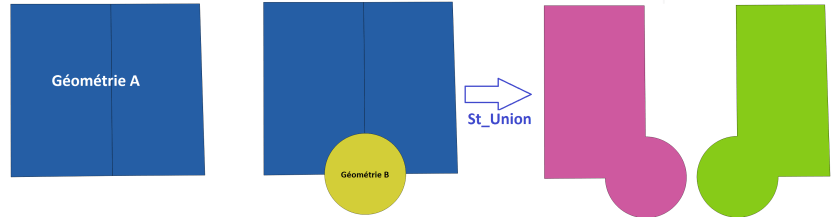If you have x geometries in A, you will have the same number of geometries in the result. It’s not the number that changes but the geometry of each entity of A that associates with the space occupied with the B geometry (ies).

This SQL function is confusing, especially in English, with the operation Union (in French Aggregation) of the ArcGis Toolset. Let’s see immediately this one to avoid any misunderstanding.

Union(Aggregation in French)

This function allows recovering all the space that is occupied by the A and B geometries:

• areas in which the geometries intersect
• areas where only A geometries are present
• areas where only B geometries are present

The following scheme shows, by imputing a layer A with two rectangles, and a layer B with a circle .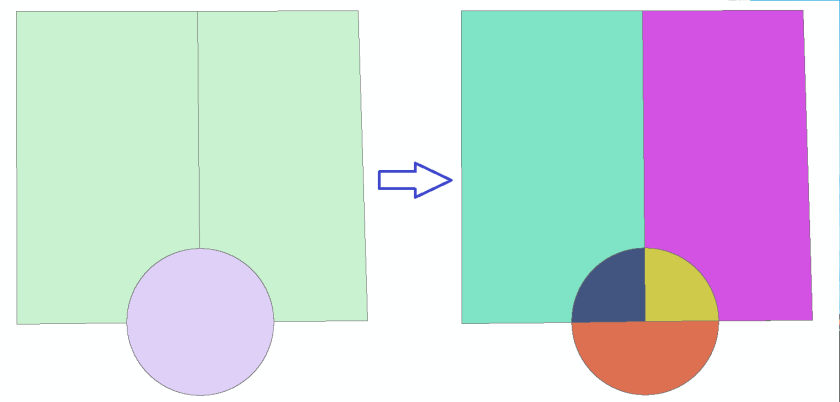The result of the union of these two layers includes 5 geometries:

• the two parts of the rectangles that do not intersect with B
• the two parts of the rectangles that intersect with the circle
• the part of the circle that does not intersect with the rectangles

We have seen that the function ST_Union keeps the same number of A geometries A in the result. With Union Toolset, there is always more geometries in the result than in the layer A, the number depending on the intersection areas of the two layers.

The sequel…

We have discussed   the three  SQL basic functions for spatial processing. In the next article we will see how to combine these basic functions to get functions such as Identity , Aggregation and Update .